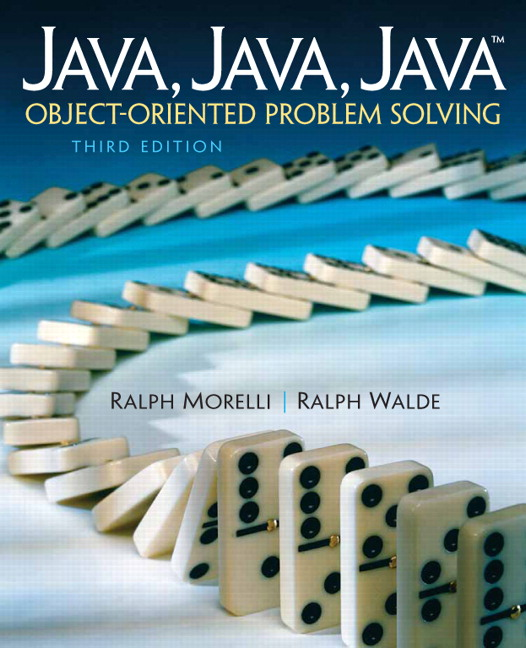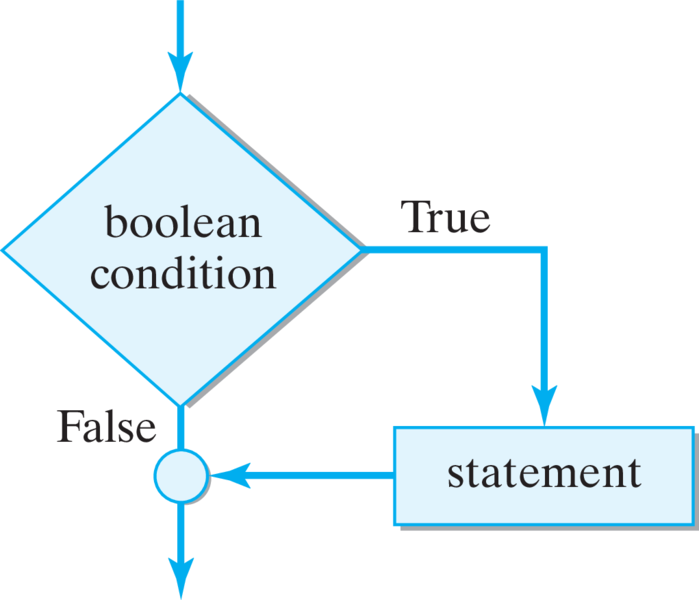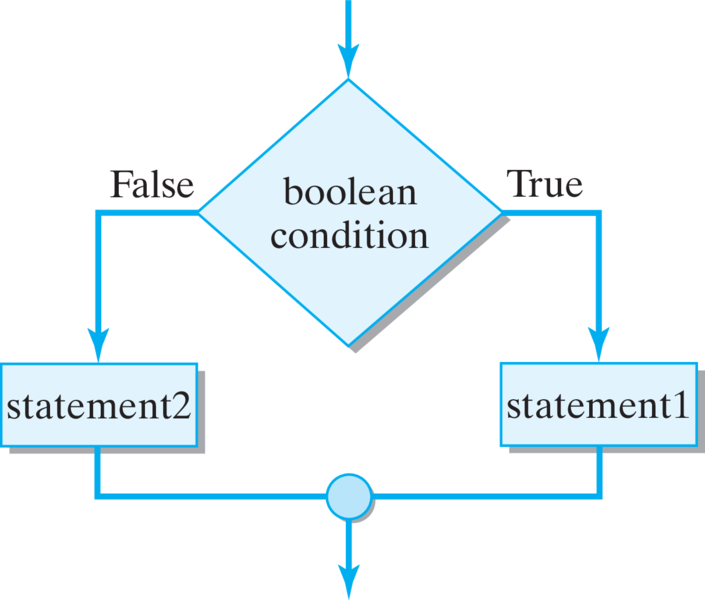# Java, Java, Java: Object-Oriented Problem Solving, 2022E

## Section3.6Flow of Control: Control Structures

We have been ignoring a couple of problems with the definition of the OneRowNim class. One problem is that we would describe a One Row Nim game as two players taking turns until there are no more sticks. An object using OneRowNim would need a way to repeatedly execute a group of statements. One command in Java that controls the repetition of a block of statements is called a while loop. We will consider it later in this section.
A second problem is with the definition of takeSticks():
public void takeSticks(int num)
{   nSticks - num;
player = 3 - player;
}

It is possible to call this method with an argument greater than 3 or less than 1. The call game.takeSticks(5) will remove 5 sticks even though the rules of One Row Nim say that you must remove 1, 2, or 3. While one might assume that the user interface should prevent the user from breaking this rule, it is a far better design if it was dealt with in OneRowNim. To do this we need a Java structure that executes different statements depending on whether the parameter is greater than 3, less than 1, or between 1 and 3. The Java if-else statement has this capability. A fuller treatment of control structures appears in Chapter 6, but in this section, we will briefly introduce a couple of simple control structures. This will enable us to write programs that take more interesting actions.

### Subsection3.6.1The Simple If Statement

A selection control structure, allows a program to select between two or more alternative paths of execution. The if statement is the most basic selection control structure in Java. Most programming languages have its equivalent.
The statement contained in the if statement can be any valid Java statement, including a compound statement. Recall from Chapter 2 that a compound statement is a set of statements contained within curly braces. The boolean expression is an expression that is either true or false. We have seen examples of boolean expressions that involve int variables, int values, and the inequality or equality operators. A method call to a method with a boolean result type is another example of a boolean expression. Given this description of if statement syntax, the following are examples of valid if statements. For readability, we usually write an if statement with its contained statement indented on the next line:
if (true)
System.out.println("Hello");
if (nSticks <= 0)
System.out.println("game is over");

The following are all examples of syntax errors involving the if statement:
if true                   // Parentheses are missing
System.out.println("Hello");
if (nSticks <= 0) return   // Semicolon missing
if ("true") return;  // "true" is not a boolean
if (true) "Hello";    // "Hello" is  not a statement

Semantically, the if statement has the following interpretation: First, the boolean condition is evaluated. If it is true, then the contained statement is executed; if it is false, then the contained statement is not executed. This is shown in Figure 3.6.2 below. The flowchart clearly shows that program flow will take one or the other of the alternative paths coming out of the diamond-shaped boolean condition box. The branch through the rectangular statement box will be taken when the boolean condition is true; otherwise the statement will be skipped.Figure 3.6.2. Flowchart of if statement.

#### Activity3.6.1.

Try the following code. Try changing the boolean isRaining to false and run again. What does it print out? Trace through the code with Show CodeLens.

### Subsection3.6.2Relational Operators

Most if statements have a boolean condition that uses relational operators like == (equal), != (not equal), <, >, <=, >=. The double equal sign == is used in if statements and other control structures to test a variable’s value. One equal sign (=) is used to assign a value to a variable like player = 1;, but two equal signs (==) are used to test the variable’s value, so that player == 1 will return true or false.
As another example, consider the definition of a getPlayerString() method for the OneRowNim class.
public String getPlayerString()
{
if (player == 1)
return "Player One"; // Exit the method
if (player == 2)
return "Player Two"; // Exit the method
return "Player error";   // Exit the method
}

The flowchart in Figure 3.6.4 shows the program flow of the entire getPlayerString() method.
It is important to note that when a return statement is executed in a method, control is returned immediately to the calling method. Thus, if player == 1  is true, the string “Player One” is returned to the calling method and the getPlayerString() method exits at this point. If it is false, then player == 2 should be true (if we have a consistent state) and the string “Player Two” should be returned and the method exited. Thus, if we have a consistent state — that is, if player has value 1 or 2—then the third return statement should never be reached.

#### Activity3.6.2.

Run the following active code a couple times until you see all the possible outputs. It prints out whether a random number is positive or equal to 0. Add another if statement that tests if it is a negative number.

### Subsection3.6.3Compound Statements inside Ifs

The following example shows the more common case where the statement contained in an if statement can be a compound statement:
if (player == 1)
{
String s = "Player One";
System.out.print(s);
System.out.println(" plays next");
System.out.println("The game is not over");
}

If player == 1 is true, then all four statements in the contained compound statement will be executed. Note here that we are declaring the local variable, s, in this block. Its scope would extend only to the end of the block. Note also that when we use a compound statement, the compound statement itself is not followed by a semicolon because it is already enclosed in braces.
Merely indenting the statements following the if clause doesn’t alter the logic of the if statement. For example, the following if statement still has only one statement in its if clause:
if (condition1)
System.out.println("One");
System.out.println("Two"); //Not part of if statement

This segment will always print “Two” because the second println() is not part of the if statement. To include it in the if statement, you must enclose both println() statements within braces:
if (condition1)
{  System.out.println("One");
System.out.println("Two");
}


### Subsection3.6.4The if-else Statement

A second version of the if statement incorporates an else clause into the structure. This allows us to execute either of two separate statements (simple or compound) as the result of one boolean expression. For example, the statement
if (player == 1)
System.out.println("Player One");
else
System.out.println("Player Two");

will print “Player One” if player == 1 is true. Otherwise, it will print “Player Two”.
As in the case of the simple if statement, the keyword if is followed by a parenthesized boolean expression, which is followed by statement1, which may be either simple or compound. If statement1 is a simple statement, then it is followed by a semicolon. The else clause follows immediately after statement1. It begins with the keyword else, which is followed by statement2, which can also be either a simple or compound statement. Note that there is no boolean expression following the else keyword. In an if-else statement, the boolean expression following the keyword if goes with both the if and else clauses.
Semantically, the if-else statement has the following interpretation: If the boolean expression is true, execute statement1; otherwise execute statement2. This interpretation is shown in Figure 3.6.7.Figure 3.6.7. If-Else Flowchart

### Subsection3.6.5The Nested if/else Multiway Selection Structure

The statements that one inserts in place of statement1 and statement2 in the if-else statement can be any executable statement, including another if statement or if-else statement. In other words, it is possible to embed one or more if-else statements inside another if-else statement, thereby creating a nested if-else control structure. As with most things, making a control structure too complex isn’t a good idea, but there is a standard nested if-else control structure that is very useful. It is known as multiway selection . As shown in Figure 3.6.8, the multiway structure is used when you want to select one and only one option from several alternatives.
Suppose we have an int variable num that will contain one of the values $$0\text{,}$$ $$1\text{,}$$ or $$2$$ unless there has been an error assigning a value to it. Suppose that we want to write code that will write out the English word for the value in num. In the example shown in Figure 3.6.8 there are three alternatives plus an error state. Here is the Java code for this example:

#### Activity3.6.3.

Run the following active code a couple times until you see all the possible outputs. It prints out the English word for a random number between 0-2. Change the random range to 4 and add another if statement to print out "three".
Note that the multiway structure has a single entry point and that only one of the four possible alternatives is executed. The code will print exactly one of the strings.
We will have many occasions to use the if-else structure. Although it does not represent a significant change, we could rewrite our takeStick() method to make use of the if-else instead of the somewhat obscure statement :
player = 3 - player;

to change the value of player from $$1$$ to $$2$$ or vice versa:
public String takeSticks(int num)
{
nSticks = nSticks - num;
if (player == 1)
player = 2;   // From 1 to 2
else
player = 1;   // From 2 to 1
}

In some respects this version of takeSticks() involves four lines of code instead of one but is simpler to understand. The if-statement tests whether the value of player is $$1\text{.}$$ If it is, the value is changed to $$2\text{.}$$ If the value of player is not $$1\text{,}$$ then the value must be $$2$$ and so the value is changed to $$1\text{.}$$ Both versions of the code will give precisely the same result, a programmer could choose to write the code either way.

#### ExercisesSelf-Study Exercises

##### 1.Flow Chart.
Consider the following method with boolean parameter.
public String getStatus(boolean isDone)
{
if (isDone)
return "Done";
else
return "Not Done";
}

Draw a flowchart for the if-else version of the getStatus() method, using the figures in this section as a guide. The if-else structure should be drawn exactly as shown in Figure 3.6.7. It should have a single entry point that leads directly to the top of a diamond-shaped box that contains a boolean condition. There should be two branches coming out of the condition box. The one going to the right is the true case, and the one going to the left is the false case. Each of these branches should contain one rectangular box, which contains the statements that would be executed in that case. The left and right branches should be connected by a circular symbol that is aligned directly under the diamond box whose conditions it connects. There should be a single exit arrow pointing directly down.
##### 2.If Debug.
Identify the errors in the following statements and fix them in the activecode below.
##### 3.getPlayerName().
Write a method named getPlayerName() that returns a String. It should return “Ann”, “Bill”, “Cal”, or “Error” when the value of player is respectively 1, 2, 3, or any other value.

### Subsection3.6.6The While Structure

A repetition or loop structure is a control structure that repeats a statement or sequence of statements in a controlled way. Many types of programming tasks require a repetition structure. Consider some examples.
• You want to add up the squares of the numbers from 1 to 100.
• You want to compute compound interest on an amount of money in a savings account with a fixed interest rate if it is kept there for $$30$$ years.
• A computer security employee wants to try every possible password in order to break into an account of a suspected spy.
• You want to have players input moves for a turn in a game until the game is over. Our OneRowNim is such an example.
We will study several different repetition structures of Java in depth in Chapter 6. We will briefly consider the while statement here so as to be able to define methods that are more powerful and more interesting. Let us write a method that solves a slight generalization of the first problem above. We will use the while statement to sum the squares of integers from $$1$$ to a number specified as a parameter of the method. Thus, the method call sumSquares(3) should return the value $$14$$ since $$1*1 + 2*2 + 3*3 = 1 + 4 + 9 = 14\text{.}$$

#### Activity3.6.4.

Run the following code to see the sum of squares method in action. Click on the CodeLens button to trace through it. Change the main method to change num and run again.
Note that in this example, the variable num gets assigned an initial value of $$1$$ before the while statement. Note also that the boolean expression num < max in parentheses after while states the condition for which we wish to continue summing squares. Finally note that the last statement in the block following the boolean expression adds $$1$$ to num–that is, this variable is updated at the end of the block.
The while statement is a loop statement in which the loop entry condition occurs before the loop body. It has the following general form:
When the while statement is executed, the loop entry condition is evaluated and if this evaluates to false, execution continues at the statement immediately after the loop body. If the loop entry condition evaluates to true, the loop body is executed and then the entry condition is evaluated again. The loop body continues to be executed until the loop entry condition evaluates to false.
To have a while statement accomplish a task, the variable or variables in the loop entry condition must be initialized correctly before the while statement and these variables must be correctly updated at the end of the loop body. We can refer to the initializer statement followed by a while statement as a while structure. We can restate the above guidelines as a design principle:
In pseudocode, the while structure would take the following form:
InitializerStatements;         // Initializer
while (loop entry condition) { // Bound test
Statements;                // Loop body
UpdaterStatements;         // Updater
}

As its form suggests, the while structure is designed so that on some conditions the loop body will never be executed. Because it tests for the loop entry condition before the loop body, it is possible that the loop body is never executed. We might say that it is designed to perform 0 or more iterations.
For example, if the method call sumSquares(-3) is executed, the loop body will be skipped, because the loop entry condition num <= max is false to begin with. No iterations will be performed, and the algorithm will simply return the value $$0\text{.}$$
Modify the definition of the sumSquares() method to define a method named sumCubes() that sums the cubes of integers from a minimum value up to a maximum value and returns that sum. sumCubes() should have two parameters that will store the minimum and maximum values. Thus the method call sumCubes(2,3) should return $$35$$ since $$2*2*2 + 3*3*3 = 8 + 27 = 35\text{.}$$
Using the sumSquares method below as a guide, define a method named sumCubes(min, max) that sums the cubes of integers from a minimum value up to a maximum value and returns that sum.